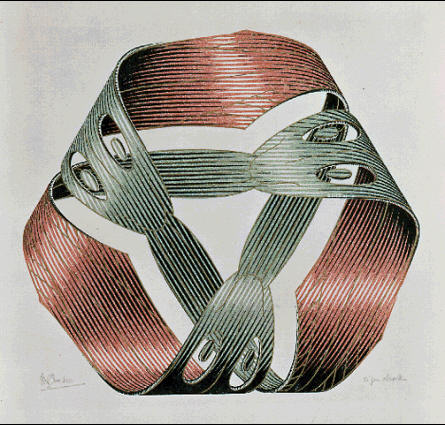Lecture slides     |     Basic equations     |      Text     |     Tools     |     Help

#### The direction of spontaneous change"The entropy of the universe tends toward a maximum"`

## The Carnot cycle provides a method for showing that the entropy can be defined as a function of the heat transferred divided by the temperature. Actually, in the early 1800s there were two applications that led to the insight that entropy is a state function that can inform us about the direction of spontaneous change. These were the steam engine and the cannon. While these may seem different they both consist of a cylinder and piston. In the steam engine, the piston moves as steam is heated inside the cylinder. The motion of the piston is converted into work. In a cannon the cannon ball acts as a piston. The explosion that occurs when gunpowder is ignited causes the "expansion", which is the exit of the cannonball from the cylinder. The common aspect that engineers wanted to understand was the efficiency of the process. By maximizing the efficiency one can also obtain the maximum work from the expansion in the steam engine. By the same reasoning, one can obtain the maximum range of the cannonball. In order to understand efficiency it is necessary to consider a cyclic process in which there is an expansion and then compression that returns the system to its initial state. One can ask the question, how much work is extracted for a given heat input? This is the efficiency. In the process of asking this question it turns out that one naturally encounters the definition of the entropy. Let's see what reasoning is employed in the Carnot cycle to obtain this result.

problem solving### Practice as you go...### Equilibration